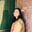Related Tags

xor
encryption
decrypt
c++

# What are XOR encryption and decryption?Umme Ammara

XOR (Exclusive-Or) is a boolean logic operation that we use widely in cryptography. The following table shows the XOR results according to different inputs:

 Input A Input B A XOR B Output 1 1 0 1 0 1 0 1 1 0 0 0

## Cryptography

It is essential to lay down the fundamentals of cryptography before diving into how XOR encryption and decryption works. Cryptography secures communication between two end-points. For example, if A wants to send a message to B, both A and B require a key to encrypt and decrypt. The steps involved are as follows:

1. The plaintext is the original text message that A wants to send to B.

2. The ciphertext is the text encrypted by A using the key.

3. B will decrypt the ciphertext back to the plaintext using the key and read the message.

The illustration below depicts the steps mentioned above:

## Example

The code below shows an example of XOR encryption and decryption using C++.

For the code below, we use the key, U to encrypt and decrypt. The plaintext, “Hello! How are you?” is passed through the XorOp function. The XOR operation takes the first bit of the plaintext and the key and returns the corresponding ciphertext. We call the XorOp function again to convert the ciphertext back to plaintext. XOR has the property of self-inverse, which makes it suitable for cryptography. The code is as follows:

#include <iostream>
#include<bits/stdc++.h>

using namespace std;

//Function for Encryption and Decryption
void XorOp (char text[], int length){
//Key
char key = 'U';
//XOR Operation
for (int i=0; i<length; i++){
text[i] = text[i]^key;
cout << text[i];
}
}

int main() {
//Plaintext
char plaintext[] = "Hello! How are you?";
int length = strlen(plaintext);

//Encryption
cout << "Ciphertext: ";
XorOp(plaintext, length);
cout << endl;

//Decryption
cout << "Plaintext: ";
XorOp(plaintext, length);
cout << endl;
return 0;
}

RELATED TAGS

xor
encryption
decrypt
c++

CONTRIBUTORUmme Ammara